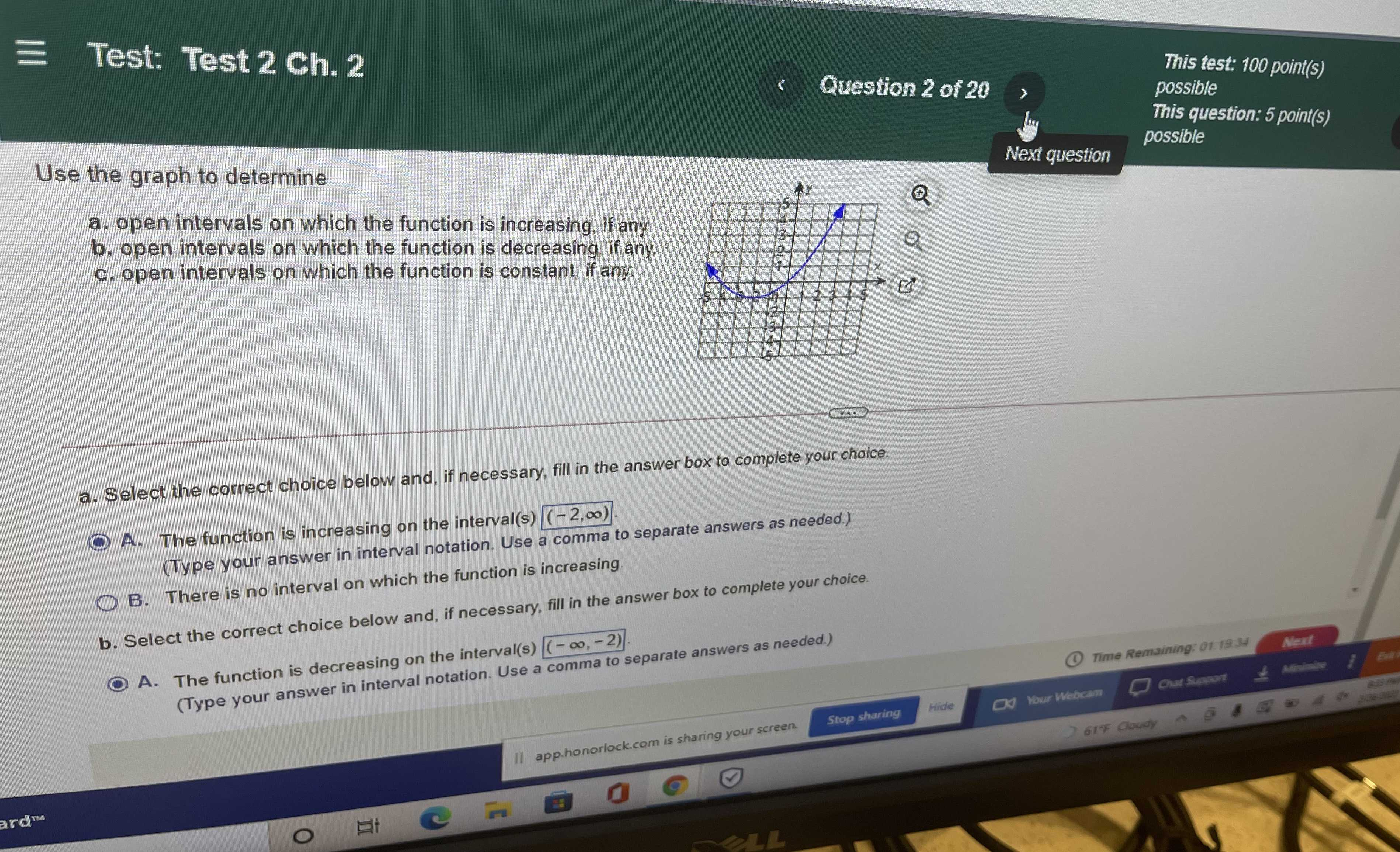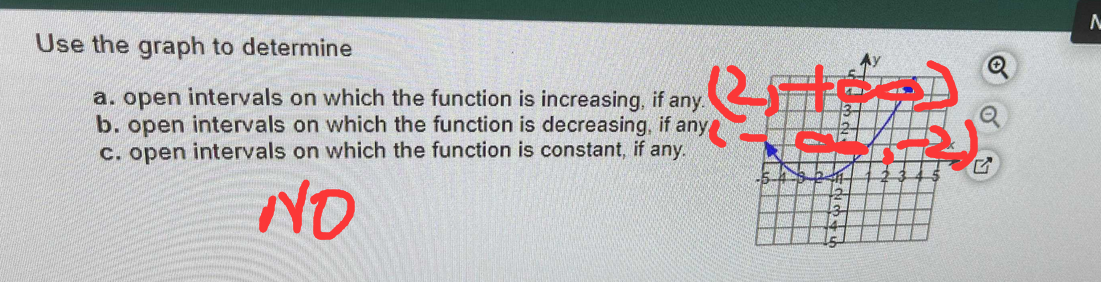### Still have math questions?

Algebra
QuestionUse the graph to determine a. open intervals on which the function is increasing, if any. b. open intervals on which the function is decreasing, if any. c. open intervals on which the function is constant, if any.

a. Select the correct choice below and, if necessary, fill in the answer box to complete your choice. A. The function is increasing on the interval(s) $$( - 2 , \infty )$$ . B. Thpe your answer in interval notation. Use a comma to separate answers as needed.) b. Select the correct choice below and, if necessary, fill in the answer box to complete your choice. A. The function is decreasing on the interval(s) $$( - \infty , - 2 )$$ . (Type your answer in interval notation. Use a comma to separate answers as needed.)